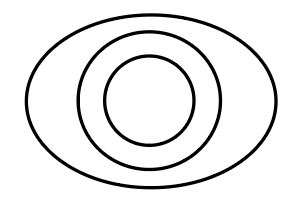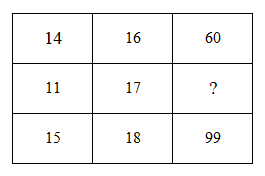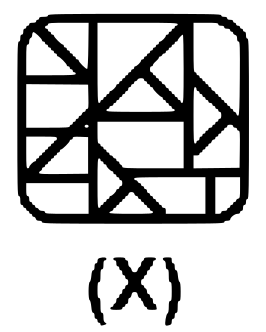# Delhi SI CPO Nov 23 Shift-1

Instructions

For the following questions answer them individually

Question 1

# Select the number-pair in which the two numbers are related in the same way asare the two numbers of the following number-pair.16: 224Question 2

# Which number will replace the question mark (?) in the following series?2430, ? , 270, 90, 30, 10Question 3

# Two statements are given, followed by three conclusions numbered I, II and III. Assuming the statementsto betrue, even if they seem to be at variance with commonly knownfacts, decide which of the conclusions logically follow(s)from the statements.Statements:All photographs are newspapers.Some photogrhaps are computers.conclusions:1. some computers are photographs11. some computers are newspaper111. some newspaper are photographsQuestion 4

# The given Venn diagram best represents the relationship between which set of classes given in the options?Question 5

# Two numbers are named as Number A and Number B. The sum of Number A,its square and its cube is 399. The sum of Number B,its square and its cube is 819. What would be the square of the number obtained by adding Number A and Number B?Question 6

# Study the given pattern carefully and select the number that can replace the question mark(?)in it.Question 7

# atan walks 15 m towards the north and then turns right and walks 23 m. Then, he turns left and walks 16 m. After that,he turns left walk 11m.Then, he turns right and walks 17 m. Then, he turns left and walks 12 m. He finally turnsright and walks 19 m. In which direction and how far is he now with reference to the starting point?Question 8

# Which number will replace the question mark (?) in the following series?82,7, 119, 142, 168, 197Question 9

# Select the option figure which is NOT embedded in the figure (X) given below(rotation is NOT allowed).Question 10

# Select the letter-cluster that can replace the question mark(?)in the given cluster series.XMT, ENA, LOH,SPO,?OR